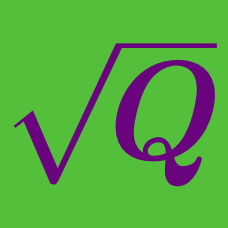Algebra

#### Challenge Quizzes

Evaluate

$( \sqrt{ 12 } - 1 ) ( \sqrt{ 12 } + 1 ).$

True or False:

For all real numbers $$a$$ and $$b,$$

$\left( \sqrt{a} + \sqrt{b} \right) ^2 = a + b .$

How many real solutions are there to

$x - 4 = 2 \sqrt{ x - 1 } ?$

The velocity (V) of an object can be obtained from the kinetic energy (KE) of the object and its mass (M) using the following formula:

$V = \sqrt{ \frac{ 2 KE } { M } }.$

If the new kinetic energy of the object (with the same mass) is 4 times the old kinetic energy, what can we say about the new velocity of the object?

What is the value of

$\sqrt{ 1 + \frac{ 16}{ 9 } } ?$

×